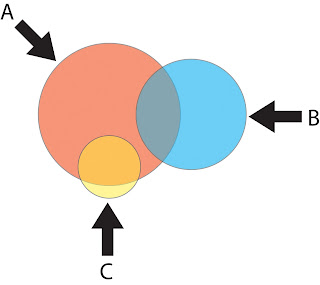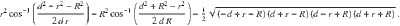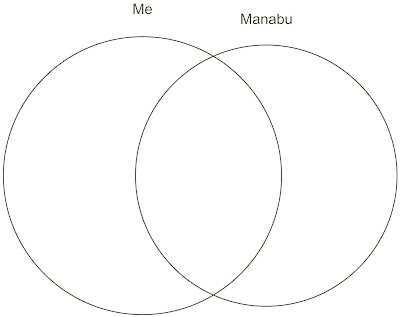## Sunday, November 09, 2008

### Who is my best friend, statistically speaking?Those of you who use the social networking site facebook may be familiar with the Friend Wheel application which takes your friends and plots them out as nodes along the edge of a circle. It then joins with a line each of your friends who are friends with each other.

My friend wheel is shown above, but who is my best friend?

I reckon I can outgeek John with this one. Surely (!) my best friend ought to be the person who has the most friends in common with me, i.e. the one with the most lines emanating from their node above. Because it would take me too long to work this out for everyone I will limit myself to those who blog: John, Manabu, Sarda and Dave.

It turns out my best friend is easily Manabu (53 friends in common), then Dave (34), Sarda (31) and John (29). However, a raw count might not be the best measure. For example if John only had 31 friends and Manabu had a thousand then surely John would be my best friend because almost all his friends would be my friends. Visually this could be represented as a Venn diagram like this:Each circle is a person and the area of the circle is the total number of friends they have. In this example I am circle A (I'm very popular, of course) and I have two friends (circles B and C) who share friends with me (represented by the area of overlap). The raw number of friends is about the same, but friend C's circle overlaps with mine much more and so between the two I would consider C to be my best friend. (Of course B and C may also share friends, but representing this would be where Venn diagrams get complicated).

So mathematically speaking my best friend would be the one whose number of friends in common with me is the greatest proportion of their total friends. Let's call this the BFI (Best Friend Index), which logically ranges from 0 (no friends in common) to 1 (all friends in common). It turns out that Manabu has the highest BFI (0.47), but what if I wanted to draw the Venn diagram reflecting this relationship accurately? This is where it gets really geeky.

First we need to draw two circles (one for me, one for Manabu) with the area being the total number of friends we each have. This is easy, right? The area is πr2, so to draw the circle we simply divide our area (number of friends) by π and then root the result. This gives us the radii needed to draw the circles.

Now it gets a bit more complicated. We know the size of the area that the overlap should be (53 friends), but this area has an odd shape that represents two segments of a circle. One for me and one for Manabu. But we cannot simply halve the total area between the two circles as the chord lengths will not match if the circles are different sizes (which they are).

This hurt my head for a bit until I found the answer here. So the area of overlap (A) is given as:Where (R) and (r) are the radii of our respective circles and (d) is the distance between the two centers - the thing we need to know. So by rearrangement we get:

Um, actually this was a bit hard for me so I posted a question on a maths forum and it seems that this equation is not rearrangeable. The only way to get d then is by an approximation method. I tried to do this using the Newton method, but this requires establishing the derivative of the above equation. Again, beyond my abilities. But after enlisting the help of a friend it turns out this too is impossible as it involves the root of a negative. So the only solution was to write my own extremely inefficient approximation method in R. Here, then, is the Venn (or is it Euler?) plot for Manabu and myself:This may all seem a bit silly, but it could easily be adapted to cover other things (e.g. favourite music and films in common etc.). If I were a more adept programmer I might have even turned this into a facebook application.